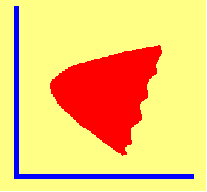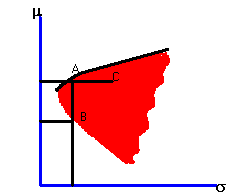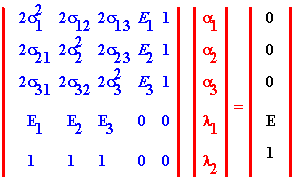4.9 THREE-FIRM CASE: APPLICATION OF MEAN VARIANCE THEORY

Graphically, our goal is to characterize the set of portfolios that lie on the efficient frontier region, extending northeast from the vertex of the investment opportunity set in Figure 4.3.

 Figure 4.3 Investment Opportunity SetRecall the statistics associated with the expected return distribution for the Three-Firm Case.

 Firm Expected Return Return Variance Covariance (i,j) (i,j) Pair Firm 1 0.229 0.924 0.063 (1,2) Firm 2 0.138 0.862 -0.582 (1,3) Firm 3 0.052 0.528 -0.359 (2,3)

Recall that the matrix representation of the three-firm problem derived in topic 4.8, and provided in table 4.1 is the following:which is more conveniently denoted as:

V A = W

Substituting the values from the three-firm problem, the V matrix becomes:

 2(0.924) 2(0.063) 2(-0.582) 0.229 1 2(0.063) 2(0.862) 2(-.359) 0.138 1 2(-0.582) 2(-0.359) 2(0.528) 0.052 1 0.229 0.138 0.052 0 0 1 1 1 0 0

Let the vector of variables being solved for be denoted as A

 a1 a2 a3 l1 l2

and the product of VA be denoted as W.

 0 0 0 E 1

The inverse of V is given by:

 0.097 -0.198 0.101 6.228 -0.46 -0.198 0.405 -0.207 -1.153 0.338 0.101 -0.207 0.105 -5.075 1.122 6.228 -1.153 -5.075 -164.523 20.339 -0.46 0.338 1.122 20.339 -2.535

Pre-multiplying W by V-1 (see topic 4.8) yields the general expression for minimum-variance portfolio weights as a function E for stocks 1,2, and 3.

Firm 1 = 6.288E - 0.460

Firm 2 = -1.153E + 0.338

Firm 3 = -5.075E + 1.122

For example, if the desired expected return is 0.14 (i.e., 14%), then substituting 0.14 for E above yields the minimum-variance portfolio weights.

 Portfolio Weights Firm 1 0.41 Firm 2 0.18 Firm 3 0.41

The next part of this chapter lets you read about properties associated with the shape of the frontier.

previous topic

next topic